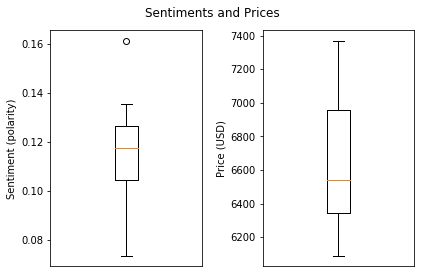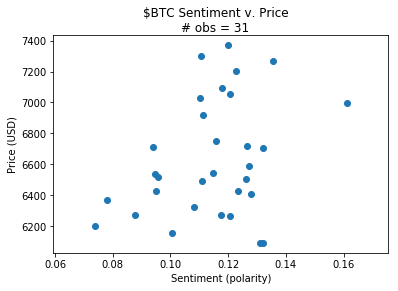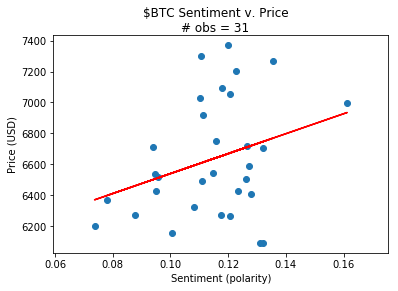# Bitcoin Sentiment-Price Correlation¶

## Inputs¶

We use the average polarity scores and the closing prices of Bitcoin from August 8 to September 7, 2018.

In :
sentiments = [0.1148378151260504,0.10054880187025132,0.1320964596633778,0.13114851605087824,0.12055378205128203,0.0737112239142696,0.08774400221116639,0.10814579489962019,0.12714464882943144,0.12790344009489915,0.12617892791127544,0.11751586287042418,0.11101584158415842,0.07804477611940298,0.0945551724137931,0.09388588503130335,0.11590802248339295,0.1265067281606077,0.11124482225656877,0.11794166666666667,0.12069146775012697,0.16124164256795834,0.11008077544426494,0.12266835016835016,0.1107218982275586,0.1354550684931507,0.11982024866785078,0.13218563411896744,0.09573690415171705,0.09506849315068491,0.12344048467569496]
prices = [6543.24,6153.41,6091.14,6091.14,6263.2,6199.6,6274.22,6323.81,6591.16,6405.71,6502.18,6269.9,6491.11,6366.13,6538.95,6708.96,6749.56,6720.6,6915.73,7091.38,7052,6998.76,7026.96,7203.46,7301.26,7270.05,7369.86,6705.03,6515.42,6426.33,6427.89]


## Graphical Analysis¶

In :
import matplotlib.pyplot as plt
%matplotlib inline

plt.figure(1)
plt.suptitle('Sentiments and Prices')

# Sentiments boxplot
plt.subplot(121)
plt.boxplot(sentiments)
plt.ylabel('Sentiment (polarity)')
plt.xticks([])

# Prices boxplot
plt.subplot(122)
plt.boxplot(prices)
plt.ylabel('Price (USD)')
plt.xticks([])

plt.tight_layout(rect=[0, 0.03, 1, 0.95])
plt.show()We see one outlier (approximately 0.16) in our sentiment dataset.

In :
plt.figure(2)
plt.scatter(x=sentiments, y=prices)
plt.suptitle('$BTC Sentiment v. Price\n# obs = 31') plt.ylabel('Price (USD)') plt.xlabel('Sentiment (polarity)') plt.show()## Regression¶ In : from scipy import stats slope, intercept, r_value, p_value, std_err = stats.linregress(x=sentiments, y=prices) plt.figure(2) plt.scatter(sentiments, prices) plt.plot(sentiments, [intercept + slope*x for x in sentiments], 'r') plt.suptitle('$BTC Sentiment v. Price\n# obs = 31')
plt.ylabel('Price (USD)')
plt.xlabel('Sentiment (polarity)')
plt.show()

print('Intercept:   {}\n'
'Slope:       {}\n'
'Correlation: {}\n'
'R-squared:   {}\n'
'p-value:     {}\n'
't-statistic: {}'
.format(intercept, slope, r_value, r_value**2, p_value, slope/std_err))Intercept:   5894.182390072815
Slope:       6453.203545109107
Correlation: 0.31132136753415407
R-squared:   0.09692099388333585
p-value:     0.08823306157092385
t-statistic: 1.7641887782716776


## Evaluation¶

We want to determine whether sentiment and price have a positive correlation. Lets test this against the null hypothesis that their correlation coefficient is zero.

Let us declare our null and alternative hypotheses:

• $\text{Hypothesis H}_0: \rho = 0$
• $\text{Hypothesis H}_A: \rho > 0$

From our above regression, we have: $\text{p-value} = Pr(T > t) = 0.0882 \text{.}$

Testing with a confidence level of 0.05, we fall outside the critical region. Thus we fail to reject our null hypothesis.

## How to Improve¶

To see the results we were expecting, we could run our inference on a dataset from a wider time interval, thereby reducing our standard error and p-value.

In the sentiment analysis phase, we could additionally tune our classifier by training with domain-specific language and vocabulary. In the case that the keyword for a currency may return Tweets totally unrelated to what we intend (such as the case with Dash or Neo), we end up factoring in junk data into our average polarity scores. Filtering out these results would inprove the validity and accuracy of our inference.